1. 长春理工大学 计算机科学与技术学院, 长春 130022;
2. 清华大学 自动化系, 北京 100084

Nonlinear compensation for optical vignetting in vision systems
DING Ying1, FAN Jingtao2, QUAN Wei1, HAN Cheng1
1. College of Computer Science and Technology, Changchun University of Science and Technology, Changchun 130022, China;
2. Department of Automation, Tsinghua University, Beijing 100084, China
Abstract: Visual inspection systems are being more and more widely applied in industrial production, but optical vignetting directly affects the imaging quality, which affects the inspection accuracy. A nonlinear compensation method for optical vignetting is given which uses the vignetting principle, to define the relative exposure of the image with coupled sampling and decoupling reconstruction to determine the camera response curve. A vignetting compensation model is then developed to eliminate the vignetting effect of the lens to improve the image quality. Tests show that the method is effective and provides excellent vision inspection quality.
Key words: information processing     vision inspection     optical vignetting effect     nonlinear compensation     relative exposure

Yu等[1-2]和Zheng等提出了一种基于查表法(look up table，LUT)来消除影像渐晕现象的算法，使用标准影像测量出数字图像灰度衰减量相对于像素位置的变化关系，建立LUT表对图像进行补偿以消除渐晕效应。李炜等提出了基于二维Gauss曲面拟合的图像灰度补偿算法，根据图像灰度分布统计特性估计二维Gauss曲面的各个参数，从而实现图像灰度补偿。但是利用该方法进行积分的计算并不够准确，同时参数估计的结果也会存在较大误差。何凯等提出利用逐行拟合的方法进行渐晕效应的复原，即使用图像相邻两行的灰度值进行图像的平滑处理，该方法可在一定程度上减轻渐晕效应影响，但当实际图像相邻两行灰度变化较大时，会出现线性条纹。何凯等又将最速下降法引入到Gauss曲面估计当中，推导出Hesse矩阵的相关系数，通过Hesse矩阵逐次迭代收敛，获得Gauss曲面的相关参数值。现有的渐晕效应补偿方法均是针对人眼的主观感受进行补偿，而没有从客观上对照度进行还原。

1 光学渐晕效应的数理模型

 $\delta P = L\mathit{\Omega }\delta \mathit{A}\cos \beta = \frac{{\rm{\pi }}}{4}{\left( {\frac{d}{z}} \right)^2}{\cos ^3}\alpha \cos \beta .$ (1)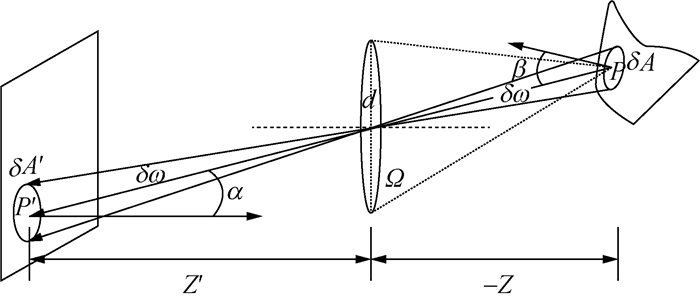图 1 物体的辐射度与图像的辐照度示意图

 $E = \frac{{\delta P}}{{\delta A'}} = \left[ {\frac{{\rm{\pi }}}{4}{{\left( {\frac{d}{{z'}}} \right)}^2}{{\cos }^4}\alpha } \right]L.$ (2)

2 渐晕效应非线性补偿方法

 ${{G'}_{\left( {x,y} \right)}} = {G_{\left( {x,y} \right)}}S\left( {{D_{\left( {x,y} \right)}}} \right).$ (3)

 ${{G'}_{\left( {x,y} \right)}} = f\left( {{f^{ - 1}}\left( {{G_{\left( {x,y} \right)}}} \right)S\left( {{D_{\left( {x,y} \right)}}} \right)} \right).$ (4)

2.1 相机响应函数

 $H = \int_0^t {E{\rm{d}}t} .$ (5)

 $H = Et.$ (6)

 ${H^ * } = \frac{H}{{{H_{\rm{s}}}}}.$ (7)

 $H_{\left( {{x_{\rm{c}}},{y_{\rm{c}}}} \right)}^ * = \frac{{{H_{\left( {{x_{\rm{c}}},{y_{\rm{c}}}} \right)}}}}{{{H_{{\rm{s}}\left( {{x_{\rm{c}}},{y_{\rm{c}}}} \right)}}}} = \frac{{{E_{\left( {{x_{\rm{c}}},{y_{\rm{c}}}} \right)}}t}}{{{E_{\left( {{x_{\rm{c}}},{y_{\rm{c}}}} \right)}}{t_{\rm{s}}}}} = \frac{t}{{{t_{\rm{s}}}}}.$ (8)

H*(xc, yc)G是一一对应的，因此，相机响应函数为G=f(H*)，相机响应函数的反函数为H*=f－1(G)。拍摄多幅图像，通过采样拟合，可获得相机的响应函数及其反函数的解析表达式。

2.2 渐晕补偿建模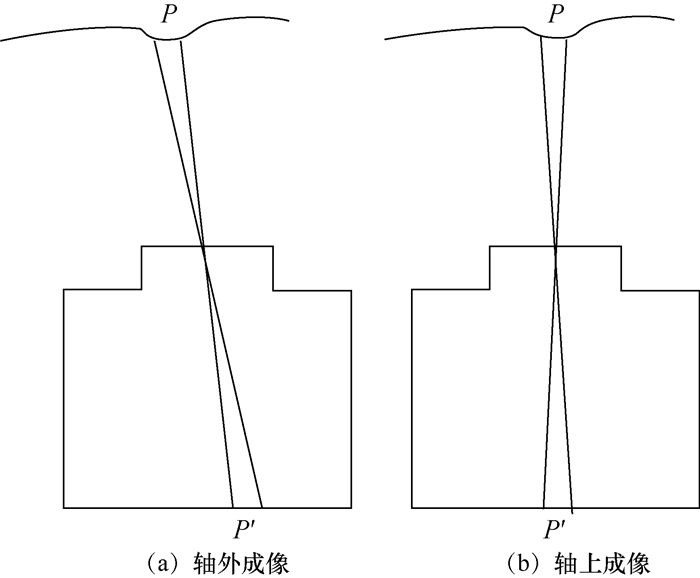图 2 相机平移成像对比图

 $\left\{ \begin{array}{l} {{G'}_{\left( {{x_i},{y_i}} \right)}} = {G_{\left( {{x_{\rm{c}}},{y_{\rm{c}}}} \right)}},\\ H_{\left( {{x_i},{y_i}} \right)}^{ * '} = H_{\left( {{x_{\rm{c}}},{y_{\rm{c}}}} \right)}^ * . \end{array} \right.$ (9)

 $\frac{{H_{\left( {{x_{\rm{c}}},{y_{\rm{c}}}} \right)}^ * }}{{H_{\left( {{x_i},{y_i}} \right)}^ * }} = \frac{{{E_{\left( {{x_{\rm{c}}},{y_{\rm{c}}}} \right)}}t}}{{{E_{\left( {{x_i},{y_i}} \right)}}t}} = \frac{{{E_{\left( {{x_{\rm{c}}},{y_{\rm{c}}}} \right)}}}}{{{E_{\left( {{x_i},{y_i}} \right)}}}}.$ (10)

 $S\left( {{D_{\left( {{x_i},{y_i}} \right)}}} \right) = \frac{{H_{\left( {{x_{\rm{c}}},{y_{\rm{c}}}} \right)}^ * }}{{H_{\left( {{x_i},{y_i}} \right)}^ * }}.$ (11)

 $H_{\left( {{x_i},{y_i}} \right)}^ * = S\left( {{D_{\left( {{x_i},{y_i}} \right)}}} \right)H_{\left( {{x_i},{y_i}} \right)}^ * .$ (12)

2.3 过曝检测

2.4 颜色空间变换

RGB是最常用的颜色空间，采用色光的色彩模式，3种色彩叠加形成不同颜色，但RGB这3个分量都包含了亮度信息，它们之间存在着很大的相关性，这种相关性不利于渐晕效应的补偿；而YCbCr是离散的颜色空间，其中Y代表图像的亮度信息，Cb和Cr分量代表颜色信息，该颜色空间将RGB分解成亮度信息和色度信息分别进行处理，且YCbCr颜色空间的Y、Cb、Cr值可以由RGB三基色经线性变换得到，计算效率高，更适用于渐晕效应补偿。

RGB空间到YCbCr空间的转换计算如下：

 $\left\{ \begin{array}{l} Y = 0.299R + 0.587G + 0.114B,\\ {\rm{Cb = 0}}{\rm{.564}}\left( {B - Y} \right) + 128,\\ {\rm{Cr = 0}}{\rm{.713}}\left( {R - Y} \right) + 128. \end{array} \right.$ (13)

 ${{Y'}_{\left( {x,y} \right)}} = f\left( {{f^{ - 1}}\left( {{Y_{\left( {x,y} \right)}}} \right)S\left( {{D_{\left( {x,y} \right)}}} \right)} \right).$ (14)
3 实验结果及分析图 3 Canon 5D Mark Ⅱ相机响应函数曲线

 $\begin{array}{l} Y = 256.1 - 181.3\exp \left( { - 0.4766X} \right) - \\ \;\;\;\;\;\;75.02\exp \left( { - 1.536X} \right). \end{array}$ (15)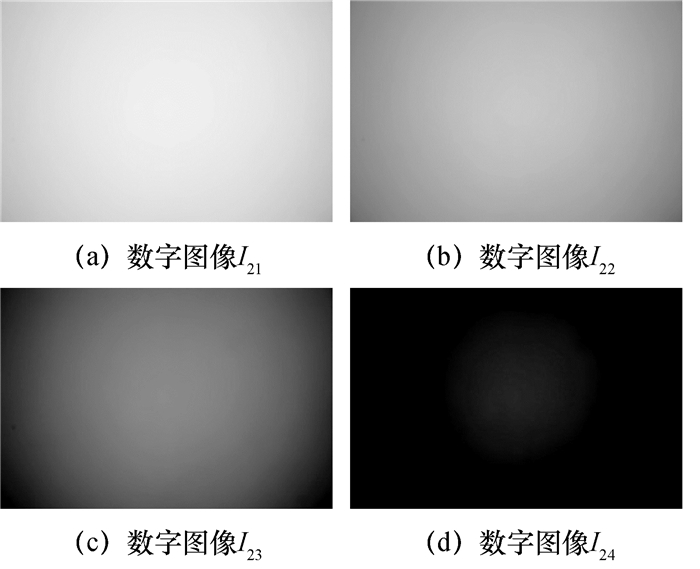图 4 40 mm焦距拍摄的数字图像I21~I24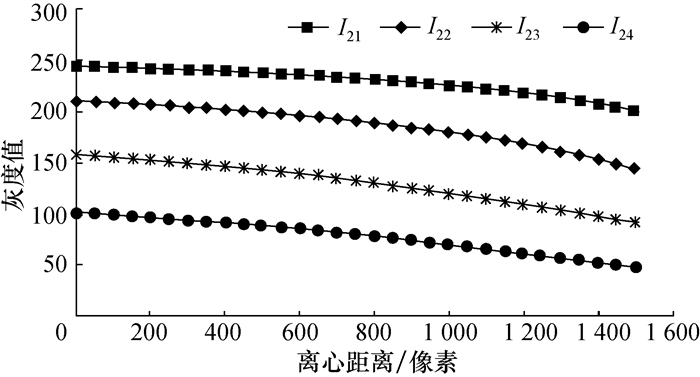图 5 数字图像I21~I24的径向灰度分布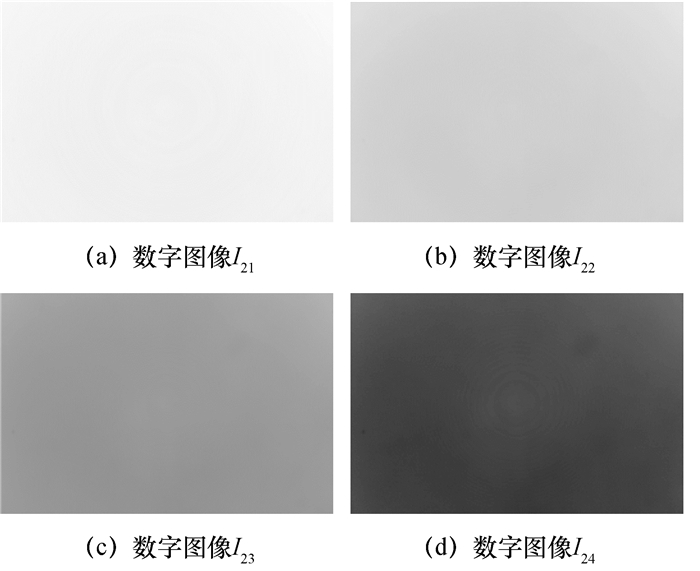图 6 数字图像I21~I24的校正结果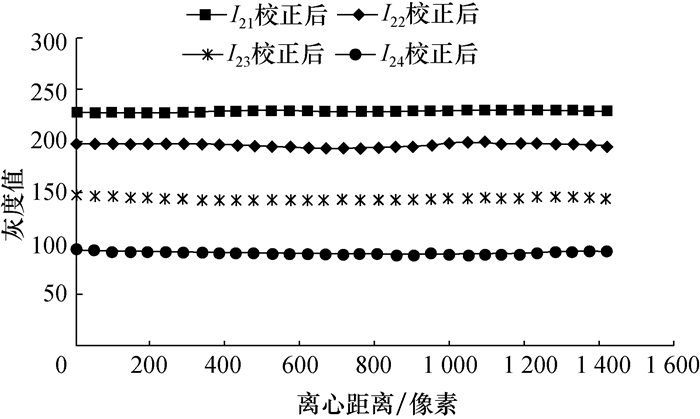图 7 数字图像I21~I24校正后的径向灰度分布

 $\sigma = \sqrt {\frac{1}{{MN}}\sum\limits_{x = 1}^M {\sum\limits_{y = 1}^N {{{\left( {{Y_{\left( {x,y} \right)}} - {Y_{\left( {{x_{\rm{c}}},{y_{\rm{c}}}} \right)}}} \right)}^2}} } } .$ (16)

 处理方法 图像I21 图像I22 图像I23 图像I24 校正前 10.30 15.68 16.02 12.32 LUT方法 3.25 9.36 11.59 9.84 本文方法 2.44 4.79 5.12 4.29

4 结论

  Yu W, Chung Y, Soh J. Vignetting distortion correction method for high quality digital imaging [C]//Proceedings of the 17th International Conference on the Pattern Recognition. Piscataway, NJ, USA: IEEE, 2004: 666-669.  Yu W. Practical anti-vignetting methods for digital cameras[J]. IEEE Transactions on Consumer Electronics, 2004, 50(4): 975–983. DOI:10.1109/TCE.2004.1362487  Zheng Y, Lin S, Kang S B. Single-image vignetting correction from gradient distribution symmetries[J]. IEEE Transactions on Pattern Analysis and Machine Intelligence, 2013, 35(6): 1480–1494. DOI:10.1109/TPAMI.2012.210  李炜, 黄心汉, 王敏. 基于二维高斯曲面拟合的图像灰度补偿算法[J]. 华中科技大学学报(自然科学版), 2004, 32(2): 43–45. LI Wei, HUANG Xinhan, WANG Min. A compensation algorithm of image based on Gaussian quadrics fitting[J]. Journal of Huazhong University of Science and Technology (Nature Science Edition), 2004, 32(2): 43–45. (in Chinese)  何凯, 赵红颖, 刘晶晶. 航空遥感影像渐晕复原方法研究[J]. 吉林大学学报(工学版), 2007, 37(6): 1447–1450. HE Kai, ZHAO Hongying, LIU Jingjing. Vignetting correction method for aviatic remote sensing image[J]. Journal of Jilin University (Engineering and Technology Edition), 2007, 37(6): 1447–1450. (in Chinese)  何凯, 唐平凡, 王成优. 基于高斯曲面拟合的影像渐晕复原方法[J]. 电子学报, 2009, 37(1): 67–71. HE Kai, TANG Pingfan, WANG Chengyou. Vignetting correction method of image based on Gaussian quadrics fitting[J]. ACTA Electronica Sinica, 2009, 37(1): 67–71. (in Chinese)  Piccinini F, Lucarelli E, Gherardi A, et al. Multi-image based method to correct vignetting effect in light microscopy images[J]. Journal of Microscopy, 2012, 248(1): 6–22. DOI:10.1111/jmi.2012.248.issue-1  Coralie C, Magali B, Arnaud D. Comparison of the vignetting effects of two identical fisheye lenses[J]. Leukos the Journal of the Illuminating Engineering Society of North America, 2012, 8(3): 181–203.  Kim S J, Pollefeys M. Robust radiometric calibration and vignetting correction[J]. IEEE Transactions on Pattern Analysis and Machine Intelligence, 2008, 30(4): 562–576. DOI:10.1109/TPAMI.2007.70732  Goldman D B, Chen J H. Vignette and exposure calibration and compensation [C]//Proceedings of the Tenth IEEE International Conference on Computer Vision. Washington DC, USA: IEEE, 2005: 2276-2288.  MENG Hongji, GUAN Wenrui, LIU Can, et al. Algorithm research of vignetting distortion correction based on near-infrared CCD thermometer [C]//International Conference on Design, Manufacturing and Mechatronics. Wuhan, China, 2015: 1290-1298.  Bergues G J, Canali L, Schurrer C, et al. Electronic interface with vignetting effect reduction for a Nikon 6B/6D autocollimator[J]. IEEE Transactions on Instrumentation and Measurement, 2015, 64(12): 3500–3509. DOI:10.1109/TIM.2015.2444263